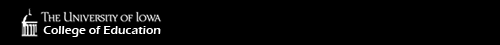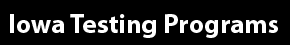Our sites: Primary Website | Login to Online Tools | Login to Bar Coding | Login to eITP# IEOC Algebra I Content Coverage

The Iowa End-of-Course assessments are designed to assess student knowledge and skills in a variety of subject areas. The test consists of 30 multiple-choice items and is designed to be administered during a single class period. The test items, which are aligned to the Common Core State Standards, reflect educator input and a rigorous quality-control process to ensure appropriateness, content accuracy, and clarity.

A summary of the content covered within the Algebra I test is presented below.

## Algebra Seeing Structure in Expressions

Topics may include performing arithmetic operations on polynomials, understanding the relationship between zeros and factors of polynomials, using polynomial identities to solve problems, and rewriting rational expressions.

## Creating Equations

Topics may include creating equations or inequalities in one or two variables, graphing equations on a coordinate axis, and solving literal equations.

## Reasoning with Equations and Inequalities

Topics may include solving linear equations and inequalities, quadratic equations, rational equations, and radical equations in one variable; solving systems of equations; and graphing linear equations and inequalities, and polynomial, rational, absolute value, exponential, and logarithmic functions.

## Functions Interpreting Functions

Topics may include using function notation and analyzing linear, quadratic, square root, cube root, piecewise-defined, polynomial, rational, exponential, and logarithmic functions.

## Building Functions

Topics may include creating functions describing relationships between quantities, using arithmetic operations on functions, composing functions, translating between explicit and recursive forms of sequences, understanding the effects of function transformations, finding inverse functions, and understanding and using the inverse relationship between exponents and logarithms to solve problems.